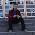### Finding the Node Position, speed and Velocity of a Node while using AODV

Finding the Node Position, speed and Velocity of a Node while using AODV

Steps
1. ~ns-2.34/aodv/aodv.h
include the following header line in aodv.h

`   1: #include<mobilenode.h>`
2.In protected scope declare the variables you would be using to store the node parameters.

`   1: double xpos;`
`   2: double ypos;`
`   3: double zpos;`
`   4: double iEnergy;`
`   5: int node_speed;`
`   6: MobileNode *iNode;`
`   7: FILE *fp;`

3.In aodv.cc initialize the declared variables.

`   1: xpos = 0.0;`
`   2: ypos = 0.0;`
`   3: zpos = 0.0;`
`   4: node_speed = 0;`
`   5: iEnergy=0.0;`
`   6: fp=fopen("pradeep.csv","w");`
`   7: MobileNode *iNode;`

4.Finally access the required functions from mobilenode.h. Paste the following lines in the AODV::forward() function

`//Code by pradeepkumar`
`/***This code retrieves node `
`position*****/`
`fprintf(fp,"Position is, X, Y, Z, Velocity is, X, Y, Z, `
`Velocity, Node Speed, Energy \n");`
`iNode = (MobileNode*) `
`(Node::get_node_by_address(index));`
`((MobileNode *) `
`iNode)->getLoc(&xpos,&ypos,&zpos);`
`//Position of %d , X, Y, `
`Z`
`fprintf(fp,"%d,%f,%f,%f,", index, xpos, ypos, zpos);`
`/***This code retrieves the nodes velocity*****/`
`iNode = (MobileNode*) `
`(Node::get_node_by_address(index));`
`((MobileNode *) `
`iNode)->getVelo(&xpos, &ypos, &zpos);`
`//Velocity of %d , X, Y, `
`Z`
`fprintf(fp,"%d,%f,%f,%f,", index, xpos, ypos, zpos);`
`/***This code retrieves the nodes speed*****/`
`iNode = (MobileNode*) `
`(Node::get_node_by_address(index));`
`node_speed = ((MobileNode *) `
`iNode)->speed();`
`iEnergy=iNode->energy_model()->energy();`
`//Velocity `
`of %d , Node Speed in m/s Energy in joules `
`fprintf(fp,"%d,%d,%f,", index, `
`node_speed,iEnergy);`

1.Hi all,

thank you

khozaimi

2.Sir,
I followed as per your instructions .But I didnt get the values of nodes position and energy .Can u help me??

Thank You

3.I get a segmentation fault when i use this code ... can u plz help ...

1.use energy model for the node configuration, you wont get segmentation fault...

4.sir i need help in adding multi interface in NS2. i want to modify mobile node architecture with multiple interface support can u help me sir

### Installing ns3 in Ubuntu 22.04 | Complete Instructions

In this post, we are going to see how to install ns-3.36.1 in Ubuntu 22.04. You can follow the video for complete details Tools used in this simulation: NS3 version ns-3.36.1  OS Used: Ubuntu 22.04 LTS Installation of NS3 (ns-3.36.1) There are some changes in the ns3 installation procedure and the dependencies. So open a terminal and issue the following commands Step 1:  Prerequisites \$ sudo apt update In the following packages, all the required dependencies are taken care and you can install all these packages for the complete use of ns3. \$ sudo apt install g++ python3 python3-dev pkg-config sqlite3 cmake python3-setuptools git qtbase5-dev qtchooser qt5-qmake qtbase5-dev-tools gir1.2-goocanvas-2.0 python3-gi python3-gi-cairo python3-pygraphviz gir1.2-gtk-3.0 ipython3 openmpi-bin openmpi-common openmpi-doc libopenmpi-dev autoconf cvs bzr unrar gsl-bin libgsl-dev libgslcblas0 wireshark tcpdump sqlite sqlite3 libsqlite3-dev  libxml2 libxml2-dev libc6-dev libc6-dev-i386 libclang-dev llvm-

### Installation of NS2 (ns-2.35) in Ubuntu 20.04

Installation of NS2 (ns-2.35) in Ubuntu 20.04 LTS Step 1: Install the basic libraries like      \$] sudo apt install build-essential autoconf automake libxmu-dev Step 2: install gcc-4.8 and g++-4.8 open the file using sudo mode \$] sudo nano /etc/apt/sources.list Include the following line deb http://in.archive.ubuntu.com/ubuntu bionic main universe \$] sudo apt update \$] sudo apt install gcc-4.8 g++-4.8 Step 3:  Unzip the ns2 packages to home folder \$] tar zxvf ns-allinone-2.35.tar.gz \$] cd ns-allinone-2.35/ns-2.35 Modify the following make files. ~ns-2.35/Makefile.in Change @CC@ to gcc-4.8 change @CXX@ to g++-4.8 ~nam-1.15/Makefile.in ~xgraph-12.2/Makefile.in ~otcl-1.14/Makefile.in Change in all places  @CC@ to gcc-4.8 @CPP@ or @CXX@ to g++-4.8 open the file: ~ns-2.35/linkstate/ls.h Change at the Line no 137  void eraseAll() { erase(baseMap::begin(), baseMap::end()); } to This void eraseAll() { this->erase(baseMap::begin(), baseMap::end()); } All changes made Step 4: Open a new termi

### Square and Sinusoidal Waveform in 8051 Microcontroller

Waveforms in 8051 Microcontroller Square Wave To create a square wave generation using Delay. let us say we want to construct a 1khz square waveform the processor instruction cycle of 8051 is 1.085microseconds so for 1khz (1milli seconds =1/1khz), is 1ms/1.085microseconds = 921.6 (this value is set to the for loop) #include <reg51.h> void delay() { int i=0; for(i=0;i<922;i++) } void main() { P0=0xff; delay(); P0=0x00; delay(); } Sine Wave generation Since sine wave is plotted in a digital device the number of samples determines the smoothness, hence in this case, more the samples, smoother is the waveform. so a lookup table is been created to get the samples. in the following examples, there are totally 36 samples are taken, starting from 0 degrees to 360 degrees with a step of 10 degrees Degrees A = 5 (1+Sin theta) Where 5 is the full scale voltage DAC = 25.6 * A 0 5 128 30 7.5 192 60 9.3 239 90 10 256 etc…like this we need to calculate for 13 samples  with a ste